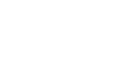Railo 4.2 Reference
Choose a function:

## Function LOG

Calculates the natural logarithm of a number. Natural
logarithms are based on the constant e (2.71828182845904).

## Example

`log(number number):number`

## Arguments

The arguments for this function are set. You can not use other arguments except the following ones.
Name Type Required Description
number number  Yes Positive real number for which to calculate the natural logarithm